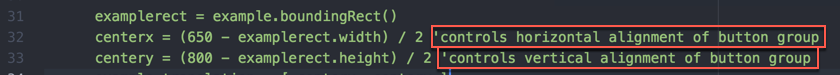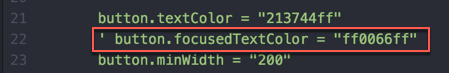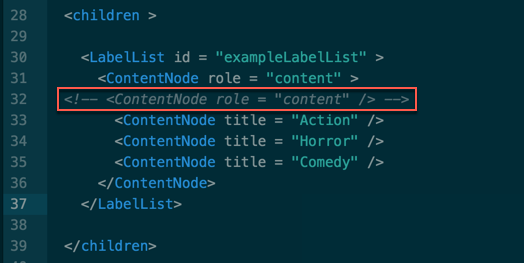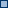# Roku SDK Documentation : Expressions, Variables, and Types

## Identifiers

Identifiers (names of variables, functions, labels, or object member functions or interfaces (appear after a ".")) have the following rules.

• must start with an alphabetic character (a – z) or the symbol "_" (underscore)
• may consist of alphabetic characters, numbers, or the symbol "_" (underscore)
• are not case sensitive
• may be of any length
• may not use a "reserved word" as the name (see Reserved Words for list of reserved words).
• if a variable: may end with an optional type designator character (\$ for string, % for integer, ! for float, # for double) (function names do not support a type designator character).

For example:

a
boy5
super_man\$

## Types

BrightScript uses dynamic typing. This means that every value also has a type determined at run time. However, BrightScript also supports declared types. This means that a variable can be made to always contain a value of a specific type. If a value is assigned to a variable which has a specific type, the type of the value assigned will be converted to the variables type, if possible. If not possible, a runtime error will result.

The following types are supported in BrightScript:

• Boolean – Either true or false.
• Integer – A 32-bit signed integer number.
• LongInteger – A 64-bit signed integer number.   This is available in firmware 7.0 or later.
• Float – A 32-bit IEEE floating point number.
• Double – A 64-bit IEEE floating point number. (Although Double is an intrinsically understood type, it is implemented internally with the roIntrinsicDouble component. This is generally hidden from the developer).
• String – A sequence of Unicode characters. Internally there are two intrinsic string states. The first is for constant strings. For example, s="astring", will create an intrinsic constant string. But once a string is used in an expression, it becomes an "roString". For example: s=s+"more", results in s becoming an "roString". If this is followed by an s2=s, s2 will be a reference to s, not a copy.
• Object – A reference to a BrightScript component. Note that if you use the "type()" function, you will not get "Object", but instead you will get the type of object. E.g. "roArray", "roAssociativeArray", "roList", "roVideoPlayer", etc.  Note that intrinsic array and associative array values correspond to roArray and roAssociativeArray components respectively.
• Function – Functions (and Subs, which are functions with void return types) are an intrinsic type. They can be stored in variables and passed to functions.
• Interface – An interface in a BrightScript component. If a "dot operator" is used on an interface type, the member must be static (since there is no object context).
• Invalid – The type invalid has only one value: invalid. It is returned in various cases, for example, when reading an array element that has never been set.
• Dynamic typing – Unless otherwise specified, a variable is dynamically typed. This means that the type is determined by the value assigned to it at assignment time. For example "1" is an integer, "2.3" is a float, "hello" is a string, etc. If a dynamically typed variable is assigned a new value, its type may change. For example: a=4 creates "a" as integer, then a = "hello", changes the variable "a" to a string. All variables are dynamically typed, unless: (a) the variable ends in a type designator character, or (b) the "As" keyword is used in a function declaration with the variable.

BrightScript 3 Compatibility

BrightScript 3 has a few changes that affect types. These are hidden from the programmer, unless the new optional "3" parameter is passed to "type()". Without this parameter, "type()" will return a BrightScript 2.1 compatible type. These are the changes:

• Non-constant strings are now stored in an "roString" component and reference counted when assigned (they were copied in version 2.1, and stored in an intrinsic type)
• Double's are now stored in an "roIntrinsicDouble" component. However, their type is still "Double". This change results in a memory use reduction most of the time (except when Doubles are actually used, then a bit more memory is used).
• Container components now store intrinsic typed values, not an auto boxed values. In other words, a= will store an integer 5 in an array, not an roInt component. This change causes less memory to be used, and execution to be faster.

Here are some examples. Note: ? is a shortcut for the "print" statement.

` BrightScript> ?type(1)  Integer  BrightScript> ?type(1.0)  Float  BrightScript> ?type("hello")  String  BrightScript> ?type(CreateObject("roList"))  roList  BrightScript> ?type(1%) Integer  BrightScript> b!=1 BrightScript> ?type(b!) Float  BrightScript> c\$="hello" BrightScript> ?type(c\$) String  BrightScript> d="hello again" BrightScript> ?type(d) String  BrightScript> d=d+d BrightScript> ?type(d) String  BrightScript> ?type(d, 3) roString  BrightScript> d=1 BrightScript> ?type(d) Integer  BrightScript> d=1.0 BrightScript> ?type(d) Float `

### In BrightScript

When the BrightScript interpreter encounters an apostrophe (') or the statement REM, it ignores all following text till the end of the line. This has multiple uses:

Used to assist in explaining code functionality, by preceding a block of code or by following a statement on the same line, e.g.When debugging

Used to disable a line. "Commenting out" code snippets is a common developer practice, e.g. say to test what the outcome is without the line:To comment out multiple lines, precede each of them with REM or apostrophe. To "block comment" big chunks of text, consider using conditional compilation.

### In XML

In the XML part of the code, in order to comment or temporarily disable a line of code, you must use <!-- comment --> as in the following example:

Note that the apostrophe DOES NOT work within the XML environment.## Literals (Constants)

Type Boolean: true, false
Type Invalid: invalid

### String Literals

Type String: String in quotes, e.g. "this is a string".

Note: The quotation mark character can be embedded in a string literal using two consecutive quotation marks.

E.g. s="""" : ? len(s), asc(s) outputs 1, 34.

This feature is available in firmware 6.2 or later.

### Numeric Literals

Type Integer: Hex integer, e.g. &HFF, or decimal integer, e.g. 255
Type Float: e.g. 2.01, 1.23456E+30, or 2!
Type Double: e.g. 1.23456789D-12 or 2.3#
Type LongInteger: Hex integer, e.g. &hFEDCBA9876543210&, or decimal integer, e.g. 9876543210&.

This feature is available in firmware 7.0 or later.

The following rules determine how integers, doubles, and floats are determined:

1. If a constant contains 10 or more digits, or if D is used in the exponent, that number is double precision. Adding a # declaration character also forces a constant to be double precision.
2. If the number is not double-precision, and if it contains a decimal point, then the number is float. If the number is expressed in exponential notation with E preceding the exponent, the number is float.
3. If neither of the above is true of the constant, then it is an integer.

### Source Literals

Type Integer: LINE_NUM – the current source line number.

### Function Literals

Type Function: e.g. MyFunction

### Array Literals

The Array Operator [ ] can be used to declare an array. It may contain literals (constants), or expressions.

Example
```myarray = [] ' empty array
myarray = [ 1, 2, 3 ] ' array of three members
myarray = [ x+5, true, 1<>2, ["a","b"] ] ' array of four members```

Arrays can be specified in multi-line form:

Example
```a = [
"able"
"baker"
]
```

or

Example
```a = [
3.1415,
2.71828
]
```

### Associative Array Literals

The { } operator can be used to define an Associative Array. It can contain literals or expressions.

Example
```aa = { }
aa = { key1: "value", key2: 55, key3: 5+3 } ```

Key names must be valid identifiers.

In firmware 7.0 or later:

Key names can be specified as string literals.

Example
`aa = { "Jane Doe": 1001, "John Doe": 1002 } `

Associative Arrays can be specified in multi-line form:

Example
```aa = {
Myfunc1: aFunction
Myval1: "the value"
}```

or

Example
```aa = {
alpha: 1,
zulu: 26
}```

## Dynamic vs. Object Types

Note on Invalid vs. Object

Certain functions that return objects can also return invalid (for example, in the case when there is no object to return). In which case, the variable accepting the result must be dynamic, since it may get "invalid" or it may get an "object".

` l=[] a\$=l.pop() `

This example will return a type mismatch (a\$ is a string, and can not contain "invalid"). Many functions that return objects can return invalid as well.

## Type Declaration Characters

A type declaration character may be used at the end of either a variable or a literal to fix its type. Variables with the same identifier but separate types are separate variables. For example, a, a\$, and a% are all different variables.

Character

Type

Examples

Notes

\$

String

A\$, STR\$

%

Integer

A%, SUM%, 125%

!

Float

A!, value!, 125!

Single-precision
#

Double

A#, distance#, 125#

Double-precision
&LongIntegerA&, ID& This is available in firmware 7.0 or later.

## Type Conversion (Promotion)

When operations are performed on one or two numbers, the result must be typed as integer, double or single-precision (float). When a +, -, or * operation is performed, the result will have the same degree of precision as the most precise operand. For example, if one operand is integer, and the other double-precision, the result will be double precision. Only when both operands are integers will a result be integer.

Division follows the same rules as +, * and -, except that it is never done at the integer level: when both operators are integers, the operation is done as float with a float result.

During a compare operation (<, >, =, etc.) the operands are converted to the same type before they are compared. The less precise type will always be converted to the more precise type.

## Effects of Type Conversions on Accuracy

When a number is converted to integer type, it is "rounded down"; i.e., the largest integer, which is not greater than the number is used. (This is the same thing that happens when the INT function is applied to the number.)

When a number is converted from double to single precision, it is "4/5 rounded" (the least significant digit is rounded up if the fractional part > =5. Otherwise, it is left unchanged).

When a single precision number is converted to double precision, only the seven most significant digits will be accurate.

## Operators

Operations in the innermost level of parentheses are performed first, and then evaluation proceeds according to the precedence in the following table. Operations on the same precedence are left associative, except for exponentiation, which is right associative.

 () Function call, or parentheses . Dot operator [ ] Array operator ^ Exponentiation – , + Negation (unary) * ,  / , MOD, \ Multiplicative operators – , + Additive operators <<, >> Integer bitshift operators <, >, = , <>, <=, >= Comparisons NOT Unary logical NOT AND Logical or bitwise OR Logical or bitwise

### Function Call Operator

The function call operator "( )" can be used to call a function. When used on a function name, function literal, or variable containing a function reference, it calls the function.

```function five() as Integer
return 5
end function

print five()
fivevar = five
print fivevar()
array = fivevar
print array()
```

### Dot Operator

The dot operator can be used on any BrightScript Component. It also has special meaning when used on any roAssociativeArray, roXMLElement or roXMLList. When used on a BrightScript Component, it refers to an interface or a member function.

Example
```i = CreateObject("roInt")
i.ifInt.SetInt(5)
i.SetInt(5)```

"ifInt" is the interface, and "SetInt" is the member function. Every member function of a BrightScript Component is part of an interface. However, specifying the interface with the dot operator is optional. If it is left out, as in the last line of the example above, each interface in the object is searched for the member function. If there is a conflict (a member function with the same name appearing in two interfaces), then the interface should be specified.

When the dot operator is used on an Associative Array, it is the same as calling the Lookup() or AddReplace() member of the AssociativeArray Object.  However the dot operator's parameters are set at compile time – they are not dynamic (unlike the Lookup() or AddReplace() functions).

Example
```aa = CreateObject("roAssociativeArray")
aa.newkey = "the value"  ' same as: aa.AddReplace("newkey", "the value")
print aa.newkey          ' same as: print aa.Lookup("newkey")```

When used for lookups, the dot operator is always case insensitive, even if ifAssociativeArray.SetModeCaseSensitive() has been called. By convention, a statement like:

`  aa.NewKey = 55`

will actually create the Associative Array entry in all lower case ("newkey").  Similarly, an AssociativeArray literal like this will also create the entry in lower case:

`  aa = { NewKey: 55 }`

To create mixed case keys, use the array operator or the ifAssociativeArray.AddReplace method:

`  aa["NewKey"] = 55`
`  aa.AddReplace("NewKey", 55)`

See the section on XML support for details on using the dot operator on xml objects.

### Array Operator

The "[ ]" operator is used to access an Array (any BrightScript Component that has an "ifArray" interface, such as roArray and roList). It can also be used as a synonym for the dot operator to access an AssociativeArray (except that the dot operator is case insensitive as described above).

```array = CreateObject("roArray", 10, true)
array = "two"
print array```
```aa = CreateObject("roAssociativeArray")
aa["newkey"] = "the value"
print aa["newkey"]
```

The "[ ]" operator takes expressions that are evaluated at runtime and so is different than the dot operator in this way.  Thus the "[ ]" operator can be used in situations where dot cannot, such as when the value of the index contains a character which is invalid in a variable name.

```aa = {}
aa.name = 1
aa["name"] = 1 ' same as previous line
aa["name with spaces"] = 2 ' cannot do this with dot operator
```

Arrays in BrightScript are one dimensional. Multi-dimensional arrays are implemented as arrays of arrays. The "[ ]" operator will automatically map a list of indexes separated by commas to the appropriate sequence of indexing. For example, the following two expressions to fetch "item" are the same:

Example
```dim array[5,5,5]
item = array
item = array[1,2,3] ```

If a multi-dimension array grows beyond its hint size the new entries are not automatically set to roArray.

### Exponentiation Operator

If x and y are integer, float or double, x^y evaluates to x raised to the power y.  Unlike other operators, exponentiation is right associative, so 2^3^2 = 2^(3^2) = 512, not (2^3)^2 = 64.

### Negation Operator

If x is integer, float or double, -x evaluates to the negation of x, and +x is equal to x.

### Multiplicative Operators

If x and y are integer, float or double, x * y evaluates to their product and x / y evaluates to their quotient.

x MOD y is the remainder when x is divided by y.

x \ y is the integer division result.  For example 7 \ 2 = 3.

x / y, x MOD y, and x \ y will all generate a runtime error if y is zero.

If x and y are integer, float or double, x+y evaluates to their arithmetic sum and x-y evaluates to their difference.  If x and y are strings, x+y is the concatenation of x and y.

### Increment and Decrement Operators

Increment (`++`) and decrement (`–`) operators are available to allow integer increment and decrement to have effect on a variable. A few examples:

`x=1`
`x++`
`' x = 2`
`x--`
`' x = 1`

`These operators are available in the 7.1 firmware version.`

### Mathematical and Bitshift Assignment Operators

The following assignment operators are available to support mathematical and bitshift operations that take a numeric operand:

• +=
• -=
• *=
• /=
• \=
• <<=
• >>=

A few examples:

`x=1`
`x+=1`
`' x = 2`
`x+=2`
`' x = 4`
`x-=1`
`' x = 3`
`x/=2`
`' x = 1.5`

`x=9`
`x\=2`
`' x = 4 (integer divide)`
`x*=3`
`' x = 12`

`x=1`
`x<<=8`
`' x = 256`
`x-=1`
`' x = 255`
`x>>=4`
`' x = 15`

`These operators are available in the 7.1 firmware version.`

### Integer Bitshift Operators

Given a number value, evaluated as an integer, and a shift value in the integer range 0..32, returns the integer value bitshifted accordingly.

A runtime error is generated if the shift value is out of range.

`Example:`
`    print 2 << 10 '= 2048`
`    print 7 >> 1 '= 3 `

Note that right shifting treats the value as an unsigned integer, e.g. &hFFFFFFFF >> 1 is equal to &h7FFFFFFF.

### Comparison Operators

This table describes the comparison operators.  All operate on either numeric values (integer, float or double) or strings.

 Operator Numeric String A = B true if A equals B true if strings A and B are identical A <> B true if A is not equal to B true if strings A and B are different A < B true if A is less than B true if string A is lexically less than string B A <= B true if (A < B) or (A = B) true if (A < B) or (A = B) A > B true if A is greater than B true if string A is lexically greater than string B A >= B true if (A > B) or (A = B) true if (A > B) or (A = B)

Note that string comparisons are case sensitive.  For example, ("one" = "One") evaluates to false.

### Logical and Bitwise Operators

AND, OR and NOT can be used both for constructing logical (Boolean) expressions and for bit manipulation. If the arguments to these operators are Boolean, then they perform a logical operation. If the arguments are numeric, they perform bitwise operations.

```x = 1 and 2 ' x is zero
y = true and false ' y is false
if a = c and not (b > 40) then print "success"```

When AND and OR are used for logical operations, the clauses are evaluated from left to right, and only the necessary amount of the expression is executed (a feature sometimes called "minimal evaluation" or "short-circuit evaluation"). For example:

`if true or func()=0 then print "ok"`

The above statement will print "ok" but will not call func, since the expression is true no matter what func returns.  On the other hand

`if false or func()=0 then print "ok"`

will call func and print ok only if func returns a value of zero.

This feature can be used to write statements such as

`if count > 0 and (total / count) > 33 then ...`

Because of minimal evaluation, this will work correctly even when count is zero, while the following similar expression would not:

`if (total / count) > 33 and count > 0 then ... ' runtime error when count = 0`

### Note on the = Operator

"=" is used for both assignment and comparison.

```a=5
if a=5 then print "a is 5"```

BrightScript does not support the use of the "=" assignment operator inside an expression (like C does). This is to eliminate the common class of bugs where a programmer meant "comparison", not "assignment". When an assignment occurs, intrinsic types (numbers, booleans, strings) are copied, but BrightScript objects (native objects, arrays, associative arrays) are reference counted.

## Attachments:commenting-example1.png (image/png)commenting-example2.png (image/png)commenting-example3.png (image/png)commenting-example3.png (image/png)